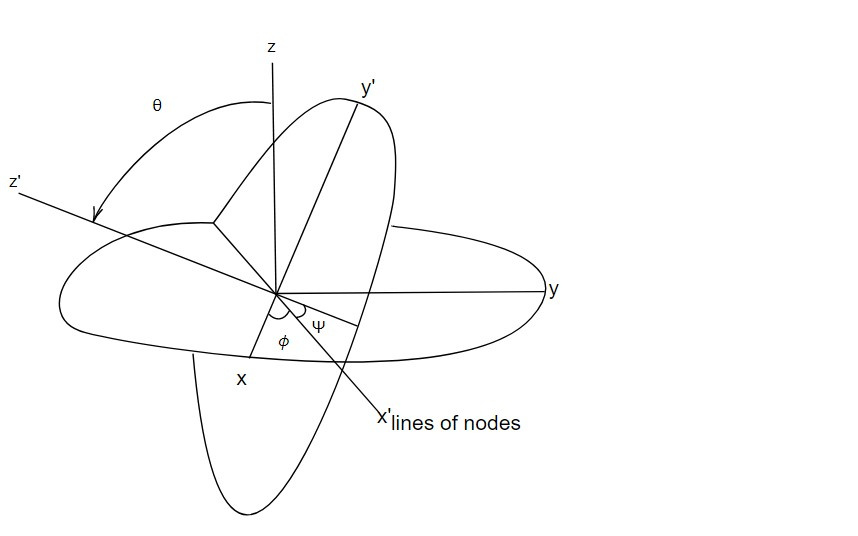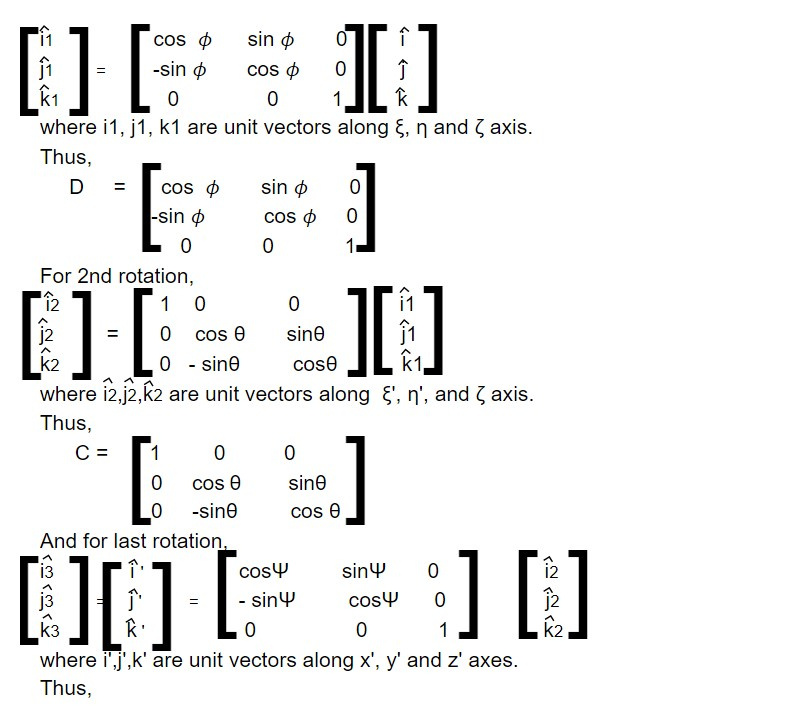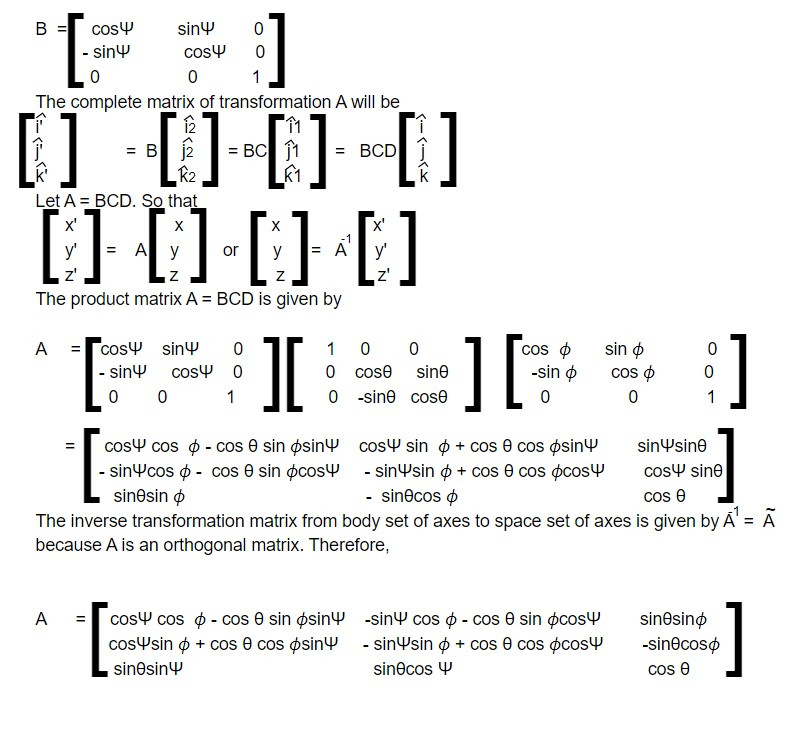# Euler's angles

The orientation of a rigid body must be specified by three independent factors in order for the associated orthogonal transformation matrix to have the determinant +1 and to be used to explain the motion of rigid bodies in the Lagrangian formulation of mechanics. Euler's angles are one such method.

The initial system of axes, xyz is rotated by an angle 𝜙 counterclockwise about the z axis, and the resultant coordinate system is labelled the ξηζ axes. In the 2nd stage the immediate axes, ξηζ are rotated about the ξ axis counterclockwise by an angle θ to produce the another intermediate set, the ξ' ,ζ'η' axes. The ξ 'axis is at the intersection of the xy and ξ' η' planes and is known as the lines of nodes. Finally the ξ' η' ζ' axes are rotated counterclockwise by an angle Ψ about the ζ' axes to produce the desired x'y'z' system of axes. The 3 angles 𝜙, θ and Ψ so formed are called Euler's angles which completely specify the orientation of the x'y'z' system relative to the xyz system. Fig given below illustrates the various stages of the sequence.The initial rotation about z can be described by a matrix D

ξ = Dx

where ξ and x stand for column matrix.

Similarly the transformation from ξ ηζ to ξ' η'ζ' can be described by a matrix C,

ξ' = Cξ,

and the last rotation to x'y'z' by a matrix B

x' = Bξ'

Hence the matrix of the complete transformation

x' = Ax

is the product of the successive matrices

A = BCD

For first rotation,This note is taken from Classical Mechanics, MSC physics, Nepal.

This note is a part of the Physics Repository.

Previous topic : Euler's theorem

Next topic : Angular momentum and Kinetic energy of motion